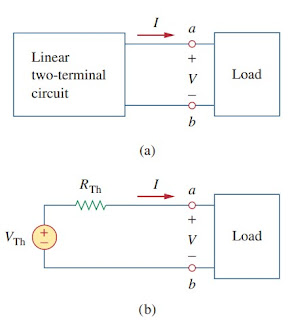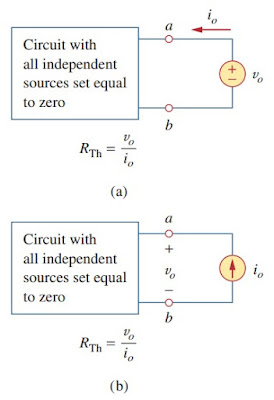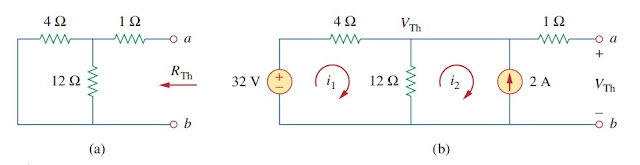omG8HI9LSKFW6lhCj8prTs0Z6lUhdOT9Jhi1Sf4m

# Thevenin's Theorem

It often occures in practice that a particulare element in a circuit is variable (usually called the load) while other elements are fixed. As a typical example, a household outlet terminal may be connected to a different appliances constituting a variable load. Each time the variable element is changed, the entire circuit has to be analyzed all over again. To avoid this problem, Thevenin's theorem provides a technique by which the fixed part of the circuit is replaced by an equivalent circuit.

This circuit analysis theorems are classified as:

## Thevenin's Theorem Theory

According to Thevenin's theorem, the linear circuit in Fig.1(a) can be replaced by that in Fig.1(b). (The load in Fig.1 may be a single resistor or another circuit.) The circuit to the left of the terminals a-b in Fig.1(b) is known as the Thevenin equivalent circuit; it was developed in 1883 by M. Leon Thevenin (1857-1926), a French telegraph engineer
Thevenin's theorem states that a linear two-terminal circuit can be replaced by an equivalent circuit consisting of a voltage source VTh in series with a resistor RTh, where VTh is the open-circuit voltage at the terminals and RTh is the input or equivalent resistance at the terminals when the independent sources are turned off.
In order to find the Thevenin equivalent voltage VTh and resistance RTh, suppose the two circuits in Figure.(1) are equivalent. Two circuits are said to be equivalent if they have the same voltage-current relation at their terminals. Let us find out what will make the two circuits in Figure.(1) are equivalent.Figure 1
If the terminals a-b are made open-circuited (by removing the load), no current flows, so that the open-circuit voltage across terminal a-b in Figure.(1a) must be equal to the voltage source VTh in Figure.(1b), since the two circuits are equivalent. Thus VTh is the open-circuit voltage across the terminals as shown in Figure.(2a); that is,Figure 2
Again, with the load disconnected and terminals a-b open-circuited, we turn off all independent sources. The input resistance (or equivalent resistance) of the dead circuit at terminals a-b in Figure.(1a) must be equal to RTh in Figure.(2b) because the two circuits are equivalent. Thus, RTh is the input resistance at terminals when the independent sources are turned off, as shown  in Figure.(2b); that is(2)
To apply this idea in finding the Thevenin resistance RTh, we need to consider two cases.
Case 1 If the network has no dependent sources, we turn off all independent sources. RTh is the input resistance of the network looking between terminals a and b, as shown in Figure.(2b)
Case 2 If the network has dependent sources, we turn off all independent sources. As with superposition, dependent sources are not to be turned off because they are controlled by circuit variables. We apply a voltage source vo at terminals a and b and determine the resulting current io. Then RTh = vo/io as shown in Figure.(3a).Figure 3
Alternatively, we may insert a current source io at terminals a-b as shown in Figure.(3b) and find the terminal voltage vo. Again RTh = vo/io. Either of the two approaches will give the same results. In either approach we may assume any value of vo and io. For example, we may use vo = 1V or io = 1A, or even use unspecified values of vo or io.

It often occurs that RTh takes a negative value. In this case, the negative resistance (v = -iR) implies that the circuit is supplying power. This is possible in a circuit with dependent sources.

Thevenin's theorem is very important in circuit analysis. It helps simplify a circuit. A large circuit may be replaced by a single independent voltage source and a single resistor. This replacement technique is a powerful tool in circuit design.

As mentioned earlier, a linear circuit with a variable load can be replaced by the Thevenin equivalent, exclusive of the load. The equivalent network behaves the same way externally as the original circuit.Figure 4
Consider a linear circuit terminated by a load RL, as shown in Figure.(4a). The current IL through the load and the voltage VL across the load are easily determined once the Thevenin equivalent of the circuit at the load's terminals is obtained, as shown in Figure.(4b). From Figure.(4b), we get(3a)(3b)
Note from Figure.(4b) that the Thevenin equivalent is a simple voltage divider, yielding VL by mere inspection.

## Thevenin's Theorem Examples

To understand better, let us review the examples below :
1.Find the Thevenin equivalent circuit shown in Figure.(5), to the left of the terminals a-b. Then find the current through RL = 6, 16, and 36 Ω
We find RTh by turning off the 32 V voltage source (replacing it with a short circuit) and the 2 A current source (replacing it with an open circuit). The circuit becomes what is shown in Figure.(6a). Thus,Figure 6
To find VTh, consider the circuit in Figure.(6b). Applying mesh analysis to the two loops, we obtain
Alternatively, it is even easier to use nodal analysis. We ignore the 1 Ω resistor since no current flows through it. At the top node, KVL gives
as obtained before. We could also use source transformation to find VTh.
The Thevenin equivalent circuit is shown in Figure.(7).
2.Find the Thevenin equivalent of the circuit in Figure.(8) at terminals a-b.
This circuit contains a dependent source, unlike the circuit in the previous example. To find RTh, we set the independent source equal to zero but leave the dependent source alone. Because of the presence of the dependent source, however, we excite the network with a voltage source vo connected to the terminals as indicated in Figure.(9a). We may set vo = 1 V to ease calculation, since the circuit is linear. Our goal is to find the current io through the terminals, and then obtain RTh = 1/io. (Alternatively, we may insert a 1 A current source, find the corresponding voltage vo, and obtain RTh = vo/1.)Figure 9
Applying mesh analysis to loop 1 in the circuit of Figure.(9a) results in(2.1)
For loops 2 and 3, applying KVL produces
To get VTh, we find voc in the circuit of Figure.(9b). Applying mesh analysis, we get(2.6)
But 4(i1-i2) = vx. Solving these equations leads to i2 = 10/3. Hence
The Thevenin equivalent is as shown in Figure.(10)
Related Posts
SHARE